# Samacheer Kalvi 10th Maths Solutions Chapter 3 Algebra Ex 3.2

## Tamilnadu Samacheer Kalvi 10th Maths Solutions Chapter 3 Algebra Ex 3.2

10th Maths Exercise 3.2 Samacheer Kalvi Question 1.
Find the GCD of the given polynomials
(i) x4 + 3x3 – x – 3, x3 + x2 – 5x + 3
(ii) x4 – 1, x3 – 11x2 + x – 11
(iii) 3x4 + 6x3 – 12x4 – 24x, 4x4 + 14x3 + 8x2 – 8x
(iv) 3x3 + 3x2 + 3x + 3, 6x3 + 12x2 + 6x + 12
Solution:
x4 + 3x3 – x – 3, x3 + x2 – 5x + 3
Let f(x) = x4 + 3x3 – x – 3
g(x) = x3 + x2 – 5x + 3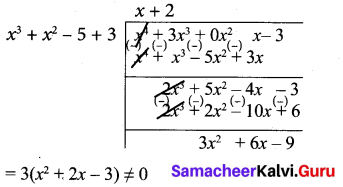Note that 3 is not a divisor of g(x). Now dividing g(x) = x3 + x2 – 5x + 3 by the new remainder x2 + 2x – 3 (leaving the constant factor 3) we get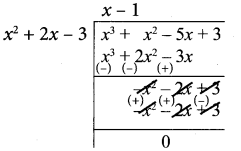Here we get zero remainder
G.C.D of (x4 + 3x3 – x – 3), (x3 + x2 – 5x + 3) is (x2 + 2x – 3)

(ii) x4 – 1, x3 – 11x2 + x – 11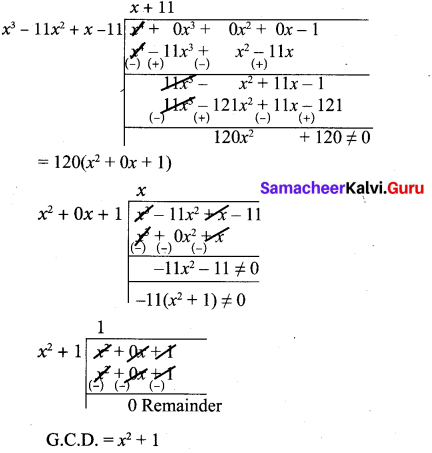(iii) 3x4 + 6x3 – 12x2 – 24x, 4x4 + 14x3 + 8x2 – 8x
4x4 + 14x3 + 8x2 – 8x = 2 (2x4 + 7x3 + 4x2 -4x)
Let us divide
(2x4 + 7x3 + 4x2 + 4x) by x4 + 2x3 – 4x2 – 8x(x3 + 4x3 + 4x) ≠ 0
Now let us divide
x4 + 2x3 – 4x2 – 8x by x3 + 4x2 + 4x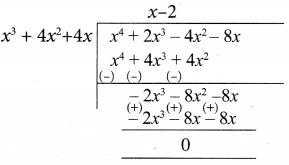∴ x3 + 4x2 + 4x is the G.C.D of 3x4 + 6x3 -12x2 – 24x, 4x4 + 14x3 + 8x2 -8x
∴ Ans x (x2 + 4x + 4)

(iv) f(x) = 3x3 + 3x2 + 3x + 3 = 3(x3 + x2 + x + 1)
g(x) = 6x3 + 12x2 + 6x + 12
= 6(x3 + 2x2 + x + 2)
= 2 × 3 (x3 + 2x2 + x + 2)
f(x) ⇒ x3 + x2 + x + 1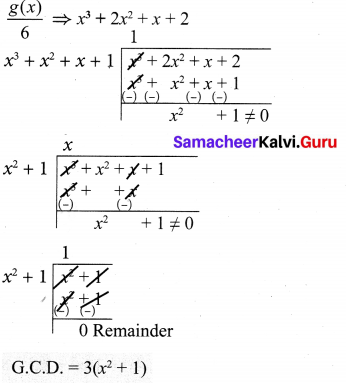The quadratic equation is an equation where the highest exponent of the variable is square. The standard form of quadratic equations is ax² + bx + c = 0.

Ex 3.2 Class 10 Samacheer Question 2.
Find the LCM of the given expressions,
(i) 4x2y, 8x3y2
(ii) -9a3b2, 12a2b2c
(iii) 16m, -12m2n2, 8n2
(iv) p2 – 3p + 2, p2 – 4
(v) 2x2 – 5x – 3, 4x2 – 36
(vi) (2x2 – 3xy)2, (4x – 6y)3, 8x3 – 27y3
Solution:
(i) 4x2y, 8x3y2
4x2y = 2 × 2 x2y
8x3y2 = 2 × 2 × 2 x3y2
L.C.M. = 2 × 2 × 2 x3y2
= 8x3 y2

(ii) -9a3b2 = -3 × 3 a3b2
12a2b2c = 2 × 3 × 2a2b2c
L.C.M. = -3 × 3 × 2 × 2 a3b2c
= -36a3b2c

(iii) 16m, -12m2n2, 8n2
16 m = 2 × 2 × 2 × 2 × m
-12m2n2 = -2 × 2 × 3 × m2n2
8n2 = 2 × 2 × 2 × n2
L.C.M.= -2 × 2 × 2 × 2 × 3 m2n2
= -48 m2n2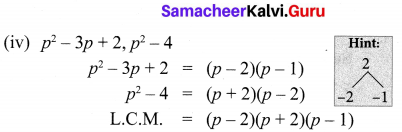(v) 2x2 – 5x – 3, 4x2 – 36
2x2 – 5x – 3 = (x – 3)(2x + 1)
4x2 – 36 = 4(x + 3)(x – 3)
L.C.M. = 4(x + 3)(x – 3)(2x + 1)

(vi) (2x2 – 3xy)2 = (x(2x – 3y))2
(4x – 6y)3 = (2(2x – 3y))3
8x3 – 27y3= (2x)3 – (3y)3
= (2x – 3y) (4x2 + 6xy + 9y2)
L.C.M. = 23 × x2 (2x – 3y)3 (4x2 + 6xy + 9y2)